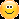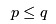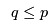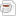# Lab 3 2020: A Cup of Java: Simplifying complex if statements and assertions.

## Introduction

The goal of this lab is to apply your knowledge about boolean expressions and propositions to the day to day programming tasks you are likely to face in the future job. If you remember from COMP102 or COMP112 (or you can learn this on the spot now), Java supports the following conditional expressions: if, while, switch that accept a boolean condition. Additionally, Java supports an assert statement that can be executed at run time and will cause your program to fail immediately if a condition evaluates to false. While you might have only used if in your assignments (if you did do COMP102 or 112) the others work in a similar fashion and require a condition. For example:

public class Test {
public boolean inOrder(int n1, int n2, int n3) {
if (n2 > n1) {
if (n3 > n2) {
return true;
} else {
return false;
}
} else if (n2 = n1) {
if (n3 = n1) {
return true;
} else {
return false;
}
} else {
return false;
}
}
}


attempts to establish the case when three numbers n1, n2, n3 are in order. Unfortunately, the program above contains a bug. See if you can discover it yourself?

ANSWER: it doesn't work properly the the case of inOrder(1,2,2) which is indeed in order.

There are two problems above. One is that the code makes too many calls, and related to this is that the code itself is too complicated to follow. Here is an example of code that is both efficient (requires few calls) and clear (try to explain how it works to your neighbour!)

public class Test {
public boolean OutOfOrder(int n1, int n2, int n3) {
return (n1 > n2) || (n2 > n3);
}
}


By applying the rules of logic you should be able to simplify complex boolean expressions (presumably produced by less careful and mathematically aware programmers) as well as check if they match the requirement in a similar manner. This lab will let you try your hand at five different examples ranging in complexity.

YOUR TASK: Is to download and edit the following five Java files using either BlueJ or any other editor of choice that supports Java. You are then required to simplify the code as much as possible by applying the first order logic skills you acquired in ENGR 123 to make the code below as short as possible while also preserving the correct behaviour. You need to submit the modified Java files (up to five) using the ENGR 123 submission system by the deadline given there, usually midnight on the day of the last lab of the week.

Please note that you are NOT allowed to use existing libraries but rather manipulate the variables below directly.

## CORE (50%) Abs and Max

### Part 1: Abs

The following function aims to return an absolute value given an integer. Simplify!

public class Abs {
public static int abs(int x) {
if(x < 0) { return -x; }
if(x >= 0) { return x; }
assert false;
return 0;
}
}


### Part 2: Max

The following function aims to return the maximum value given two integers. Simplify!

public class Max {
// Should return the largest of either a or b.
public static int max(int a, int b) {
if(a < b || a == b) {
return b;
} else {
return a;
}
}
}


## COMPLETION (30%) Point and Rectangle

### Part 3: Point

The following function aims to compare two Points with fields x and y for equality. Simplify!

public class Point {
private int x;
private int y;

public Point(int x, int y) {
this.x = x;
this.y = y;
}

public boolean equals(Object o) {
if(o instanceof Point) {
Point c = (Point) o;
if(!(c.x = this.x && c.y = this.y)) {
return false;
} else {
return true;
}
}
return false;
}

}


### Part 4: Rectangle, the contains method

The following function aims to check if a Rectangle contains a Point. Simplify by using calls to InOrder. Note this isn't the most efficient strategy in terms of the number of operations, but your code should be much more readable than the original.

// Construct a rectangle from two arbitrary points
public class Rectangle {
private int x1;
private int y1;
private int x2;
private int y2;

public Rectangle(int x1, int y1, int x2, int y2) {
this.x1 = x1;
this.y1 = y1;
this.x2 = x2;
this.y2 = y2;
}

public boolean contains(int x, int y) {
if(x1 <= x2) {
if(x1 <= x && x <= x2) {
if(y1 <= y2) {
return y1 <= y && y <= y2;
} else {
return y2 <= y && y <= y1;
}
}
} else {
if(x2 <= x && x <= x1) {
if(y1 <= y2) {
return y1 <= y && y <= y2;
} else {
return y2 <= y && y <= y1;
}
}
}

return false;
}
}


## CHALLENGE (20%) InOrder x2 and Inbetween

### Part 5a: InOrder using OutOfOrder

Simplify the InOrder code by making a single call to OutofOrder.

### Part 5b: Using rules of logic

Use the code for OutOfOrder and the rules of logic , to make InOrder as simple as OutOfOrder.

### Part 5c: InBetween

The command InBetween determines when m is in between p and q, where p and q are not necessarily in order i.e.andare both acceptable as long as m is between for one of them.

public class Test {
public boolean InBetween(int m, int p, int q) {
return ((m-p)(q-m)>=0);
}
}


This is very efficient, it only uses two subtractions, one multiplication and one inequality, four operations all up. This is very efficient, but it isn't immediately obvious that it works!

Use calls to InOrder to get the same result with much clearer code, but include a one line comment explaining why this strategy is much more inefficient.

## Files

Topic attachments
I Attachment Action Size Date Who Commentjava Abs.java manage 140 bytes 19 Jul 2016 - 09:27 Main.sarcherjava InOrder.java manage 1 K 26 Jul 2016 - 08:15 Main.sarcherjava Max.java manage 186 bytes 19 Jul 2016 - 09:27 Main.sarcherjava Point.java manage 352 bytes 19 Jul 2016 - 09:28 Main.sarcherjava Rectangle.java manage 981 bytes 25 Jul 2016 - 17:06 Main.sarcher